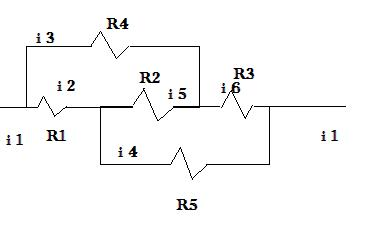# Kirchoff's Laws

Liquidxlax

## Homework Statement

don't mind my cruddy drawing :PR1 to R3 = 1000 Ω

R4 to R5 = 2000 Ω

i1 = 1mA= 1x10-3A

Find the current through each resistor.

## Homework Equations

parallel Rp = (sum)(1/Ri)-1

series Rs = (sum)(Ri))

ΔV=iR

## The Attempt at a Solution

i'm not sure of the best way to star this one because it is a little awkward to me. I thought the best way to do it would be to find Equivalent Resistance, but I have no idea how to do that with this geometry.

i know current at the start equals the end. each loop from start to finish ends in 0 potential

0 = ΔV -i2R1 - i5R2 - i6R3

0 = ΔV -i2R1 - i4R5

0= ΔV - i3R4 - i6R3

i1 = i2 + i3

thanks

Mentor

## Homework Statement

don't mind my cruddy drawing :PR1 to R3 = 1000 Ω

R4 to R5 = 2000 Ω

i1 = 1mA= 1x10-3A

Find the current through each resistor.

## Homework Equations

parallel Rp = (sum)(1/Ri)-1

series Rs = (sum)(Ri))

ΔV=iR

## The Attempt at a Solution

i'm not sure of the best way to star this one because it is a little awkward to me. I thought the best way to do it would be to find Equivalent Resistance, but I have no idea how to do that with this geometry.

i know current at the start equals the end. each loop from start to finish ends in 0 potential

0 = ΔV -i2R1 - i5R2 - i6R3

0 = ΔV -i2R1 - i4R5

0= ΔV - i3R4 - i6R3

i1 = i2 + i3

thanks

When in doubt, I just start writing KCL equations and then solve them for the unknowns.

So in this circuit, I'd draw ground at the right node, put an unknown voltage V at the left node, and write KCL equations for the middle 2 nodes. Solve away, and see if that gets you what you want...

Liquidxlax
if i'm thinking of the right nodes... how can you write an equation for the middle? you have to include the outer ones. i wrote out every equation i could possibly think of.

I'm to tired right now to fuss over the little things. just imagine the numbers are the currents and the nodes are a b c d

@ a 1 = 2 + 3

@ b 2 = 4 + 5

@ c 6 = 5 + 3

@ d 1 = 6 + 4

6+4 = 3+2

6+4= 6-5 +2

4 = 2-5

kind aggravated with this one

Mentor
if i'm thinking of the right nodes... how can you write an equation for the middle? you have to include the outer ones. i wrote out every equation i could possibly think of.

I'm to tired right now to fuss over the little things. just imagine the numbers are the currents and the nodes are a b c d

@ a 1 = 2 + 3

@ b 2 = 4 + 5

@ c 6 = 5 + 3

@ d 1 = 6 + 4

6+4 = 3+2

6+4= 6-5 +2

4 = 2-5

kind aggravated with this one

I'm not understanding your post. The KCL equations will be one per node, and will be in the form of a sum of currents. Each current will generally be expressed as a voltage difference between 2 nodes divided by the resistance between those two nodes.

Liquidxlax
I'm not understanding your post. The KCL equations will be one per node, and will be in the form of a sum of currents. Each current will generally be expressed as a voltage difference between 2 nodes divided by the resistance between those two nodes.

i guess i will redraw my picture and label the nodes. i know what you mean, by kcl. the first node would be

current 1 = current 2 + current 3

Mentor
i guess i will redraw my picture and label the nodes. i know what you mean, by kcl. the first node would be

current 1 = current 2 + current 3

I prefer to write my KCL equations as the sum of all currents leaving a node is zero. But that's up to personal preference.

Liquidxlax
I prefer to write my KCL equations as the sum of all currents leaving a node is zero. But that's up to personal preference.

okay but at the nodes 3 and 4 with current i5 and i4, do those split up 2 ways or do they just continue to the end of the circuit?